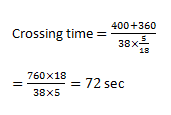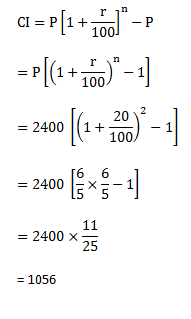# SSC Quantitative Aptitude Questions (Day-45)

Dear Aspirants, you can find the Quantitative Aptitude questions with detailed explanations for the SSC exams. Nowadays the competitive level of the exam has been increasing consistently. Due to the great demand for the government job, the level of the toughness reached greater. Candidates have to enhance the preparation process in order to drive in the right path. It doesn’t need to clear the prescribed cutoff. You must have to score good marks more than the cut off marks to get into the final provisional list. Here we have updating the Quantitative Aptitude questions with detailed explanations on a daily basis. You can practice with us and measure your level of preparation. According to that you can sculpt yourself in a proper way. SSC aspirants kindly make use of it and grab your success in your career.

Start Quiz

1) If 3P = 5Q = 15R, then what is P : Q : R?

(a) 5 : 3 :  4

(b) 5 : 3 : 1

(c) 3 : 5 : 15

(d) 15 : 5 : 3

2) What is the average of the cubes of the first 13 natural numbers?

(a) 196

(b) 364

(c) 485

(d) 637

3) The average of 50 results was calculated as 30 but later it was found that while calculating, 73 was taken as 33 by mistake, then what will be the correct average?

(a) 29.2

(b) 30.8

(c) 31.6

(d) 34

4) By selling a table for Rs. 2700 a man gets 10% loss, and then at what price (in Rs.) should he sell to gain 33
1/3%?

(a) 3000

(b) 3300

(c) 3600

(d) 4000

5) A trader sells two articles for Rs. 14784 each. If he gains 12% on one and losses 12% on other, then what is the value (in Rs) of the loss?

(a) 300

(b) 368

(c) 432

(d) 498

6) If the length of a rectangle is decreased by 20% and breadth is decreased by 40%, then what will be the percentage decrease in the area of the rectangle?

(a) 48

(b) 52

(c) 60

(d) 40

7) If the radius of sphere is decreased by 10%, then by what percent volume of sphere will decrease?

(a) 30

(b) 27.1

(c) 29.3

(d) 28.5

8) 45 pillars are standing in a line such that distance between any two consecutive pillars is same. A car travelling with uniform speed of 72 km/h takes 18 seconds to reach from 1st pole to 10th pole. What is the distance between 10th and 31st pole (in metres)?

(a) 800

(b) 820

(c) 840

(d) 910

9) Two trains are moving in the same direction at speed of 54 km/h and 92 km/h, their lengths are 400 m and 360 m respectively. What is the time taken (in seconds) by faster train to cross the slower train?

(a) 60

(b) 72

(c) 81

(d) 90

10) What is the compound interest (in Rs) on Rs 2400 at the rate of 20% per annum compounded yearly for 2 years?

(a) 960

(b) 1024

(c) 1056

(d) 1120

3P = 5Q = 15R

P : Q = 5 : 3

Q : R = 15 : 5

OR Q : R = 3 : 1

P : Q : R = 5 : 3 : 1

The sum of the cubes of first n natural no.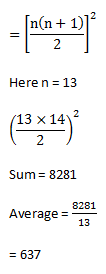Calculated sum = 50 × 30

= 1500

New sum = 1500 + 40

= 1540

New average = 1540/50

= 30.8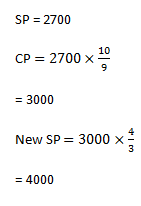Let CP of first articles = x

CP of second articles = y

Given,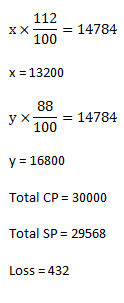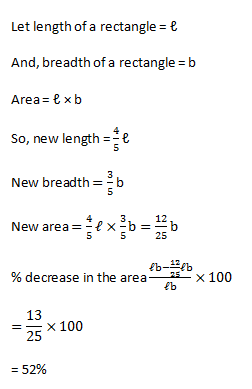Volume = 1000 : 729

% decrease in volume = 271/1000 × 100

= 27.1%

Distance between 1st pole to 10th pole (d) = v × t

= 72 × 5/18 × 18

= 360 m

Distance between 9 poles= 360 m

Distance between 2 poles = 360/9

= 40 m

Distance between 21 poles [10th and 31st pole]

= 21 × 40

= 840 m

Relative speed in same direction = 92-54

= 38 km/h